# C - Pointers and Strings

C ProgrammingIn this tutorial we will learn to store strings using pointers in C programming language.

We know that a string is a sequence of characters which we save in an array. And in C programming language the `\0` null character marks the end of a string.

## Creating a string

In the following example we are creating a string `str` using `char` character array of size 6.

``````char str = "Hello";
``````

The above string can be represented in memory as follows.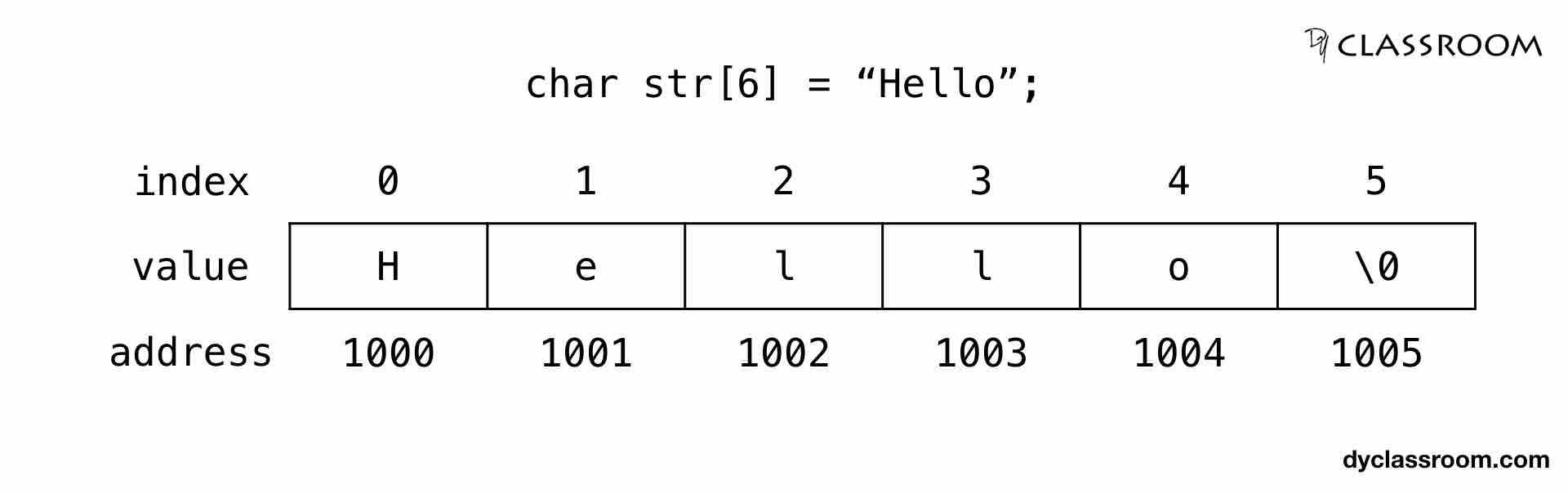Each character in the string `str` takes 1 byte of memory space.

## Creating a pointer for the string

The variable name of the string `str` holds the address of the first element of the array i.e., it points at the starting memory address.

So, we can create a character pointer `ptr` and store the address of the string `str` variable in it. This way, ptr will point at the string str.

In the following code we are assigning the address of the string `str` to the pointer `ptr`.

``````char *ptr = str;
``````

We can represent the character pointer variable `ptr` as follows.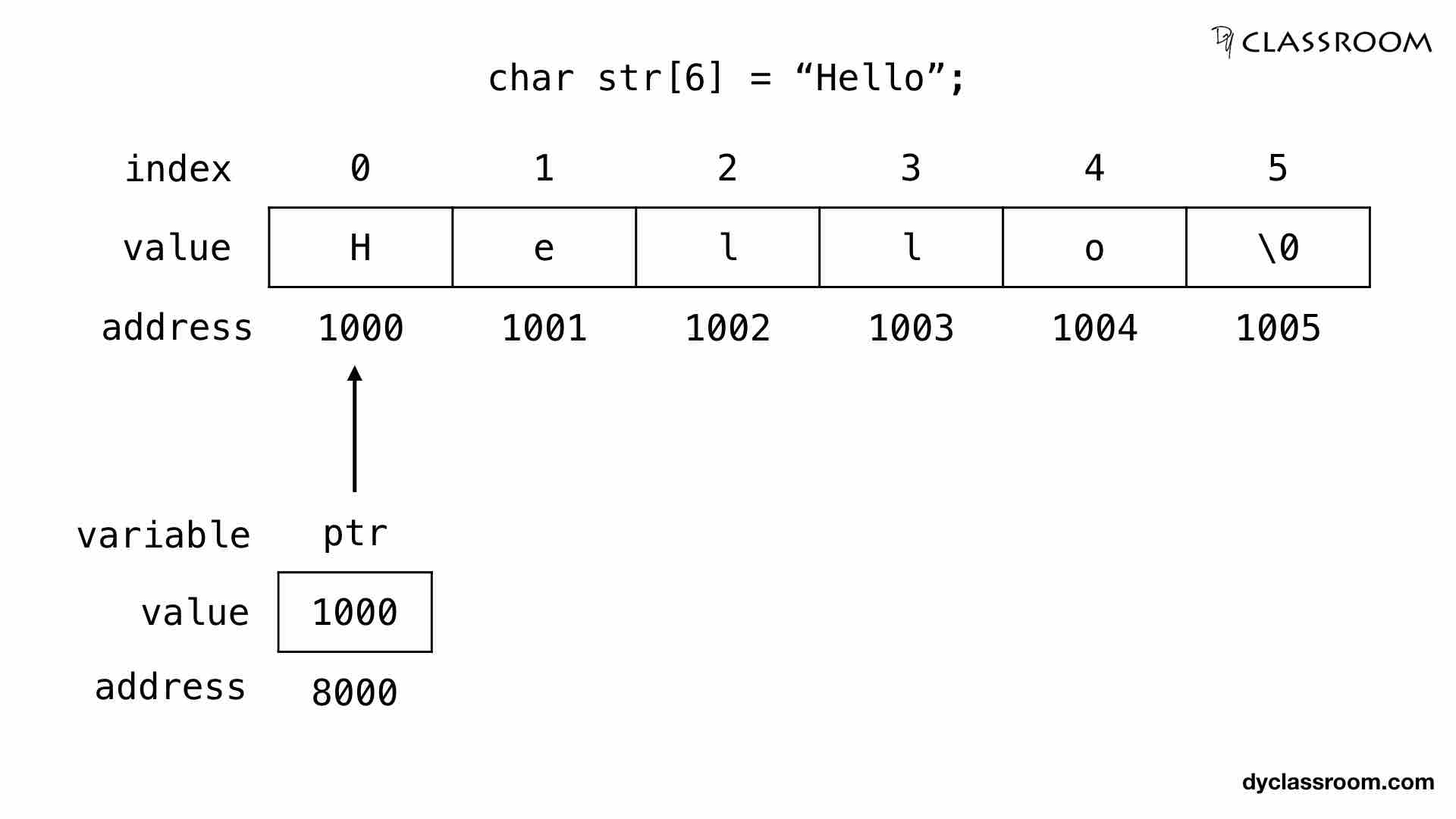The pointer variable `ptr` is allocated memory address 8000 and it holds the address of the string variable `str` i.e., 1000.

## Accessing string via pointer

To access and print the elements of the string we can use a loop and check for the `\0` null character.

In the following example we are using `while` loop to print the characters of the string variable `str`.

``````#include <stdio.h>

int main(void) {

// string variable
char str = "Hello";

// pointer variable
char *ptr = str;

// print the string
while(*ptr != '\0') {
printf("%c", *ptr);

// move the ptr pointer to the next memory location
ptr++;
}

return 0;
}
``````

## Using pointer to store string

We can achieve the same result by creating a character pointer that points at a string value stored at some memory location.

In the following example we are using character pointer variable `strPtr` to store string value.

``````#include <stdio.h>

int main(void) {

// pointer variable to store string
char *strPtr = "Hello";

// temporary pointer variable
char *t = strPtr;

// print the string
while(*t != '\0') {
printf("%c", *t);

// move the t pointer to the next memory location
t++;
}

return 0;
}
``````

Note! In the above code we are using another character pointer `t` to print the characters of the string as because we don't want to lose the starting address of the string "Hello" which is saved in pointer variable `strPtr`.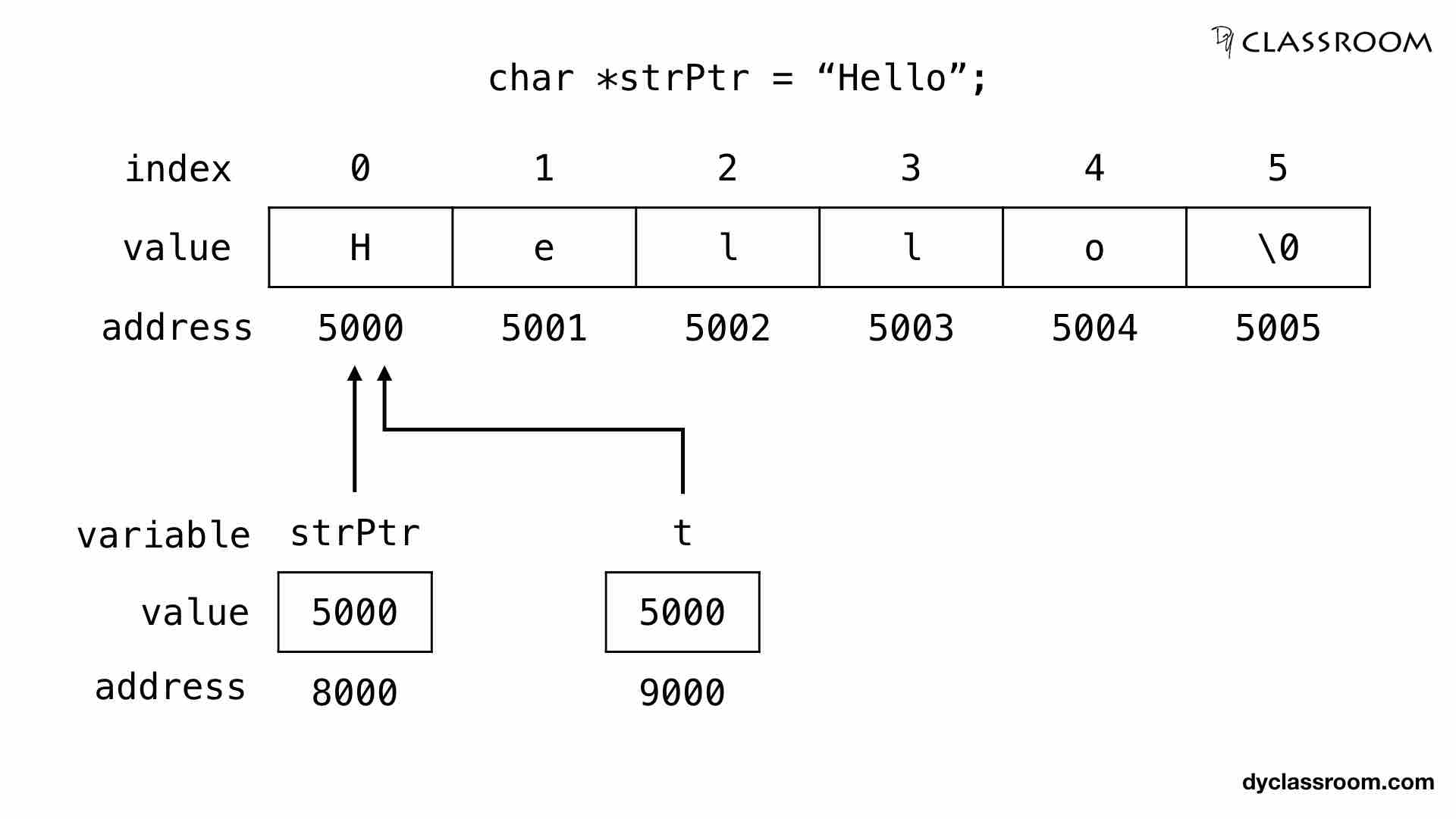In the above image the string "Hello" is saved in the memory location 5000 to 5005.

The pointer variable `strPtr` is at memory location 8000 and is pointing at the string address 5000.

The temporary variable is also assigned the address of the string so, it too holds the value 5000 and points at the starting memory location of the string "Hello".

## Array of strings

We can create a two dimensional array and save multiple strings in it.

For example, in the given code we are storing 4 cities name in a string array `city`.

``````char city = {
"Chennai",
"Kolkata",
"Mumbai",
"New Delhi"
};
``````

We can represent the city array as follows.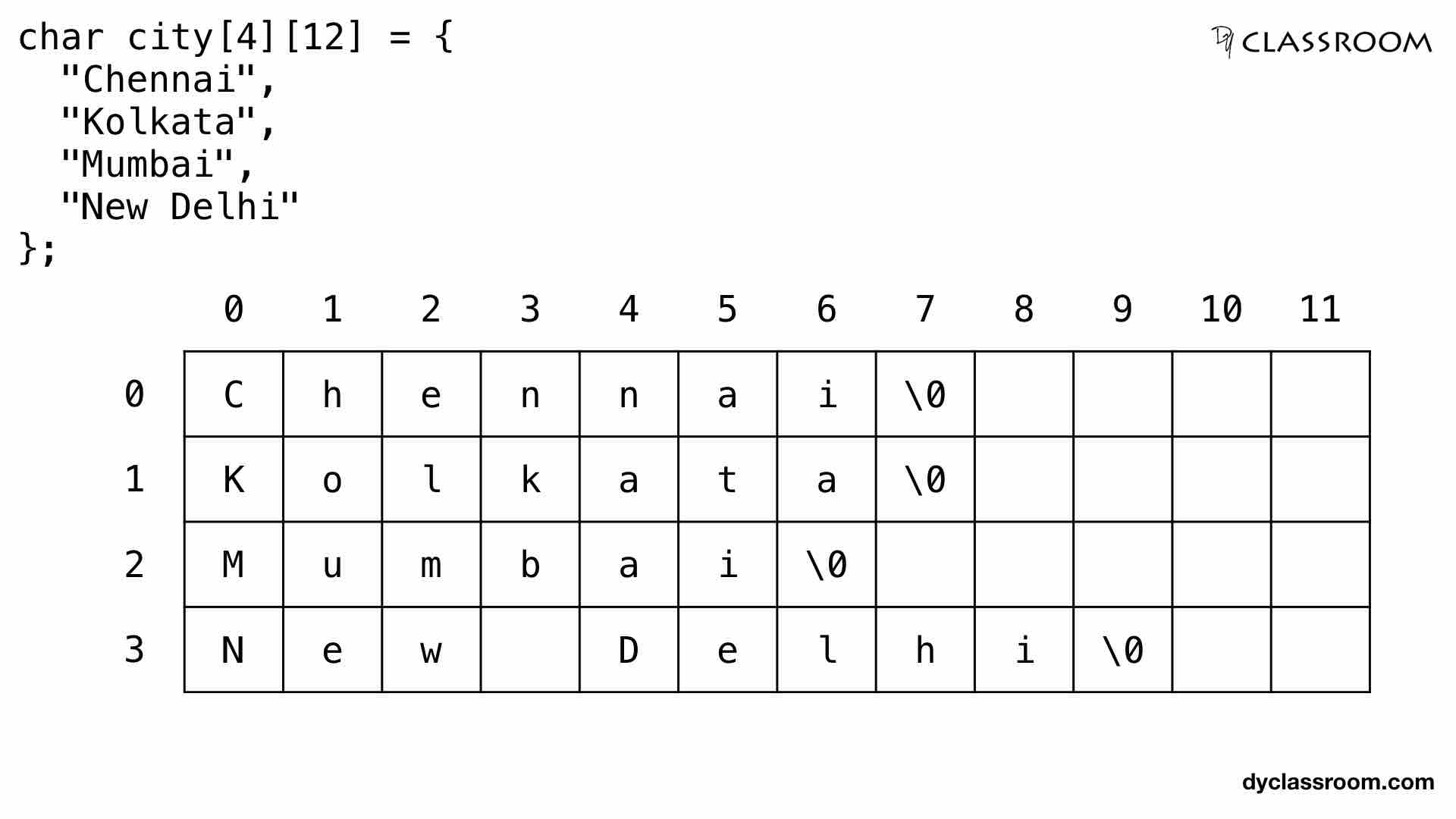The problem with this approach is that we are allocating 4x12 = 48 bytes memory to the city array and we are only using 33 bytes.

We can save those unused memory spaces by using pointers as shown below.

``````char *cityPtr = {
"Chennai",
"Kolkata",
"Mumbai",
"New Delhi"
};
``````

In the above code we are creating an array of character pointer `cityPtr` of size 4 to store the name of the four cities.

We can represent the array of pointers as follows.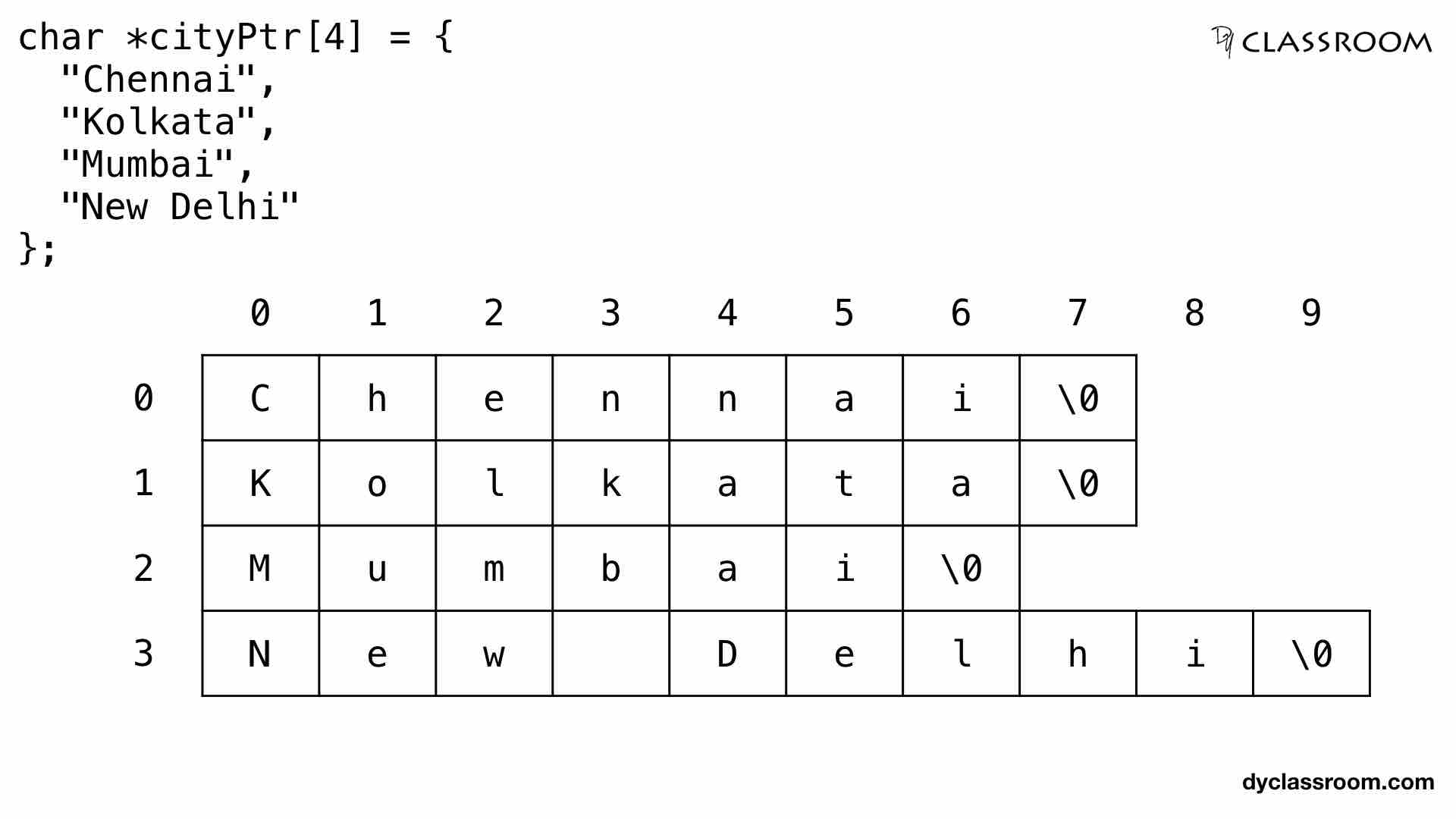The above array of pointers can be represented in memory as follows.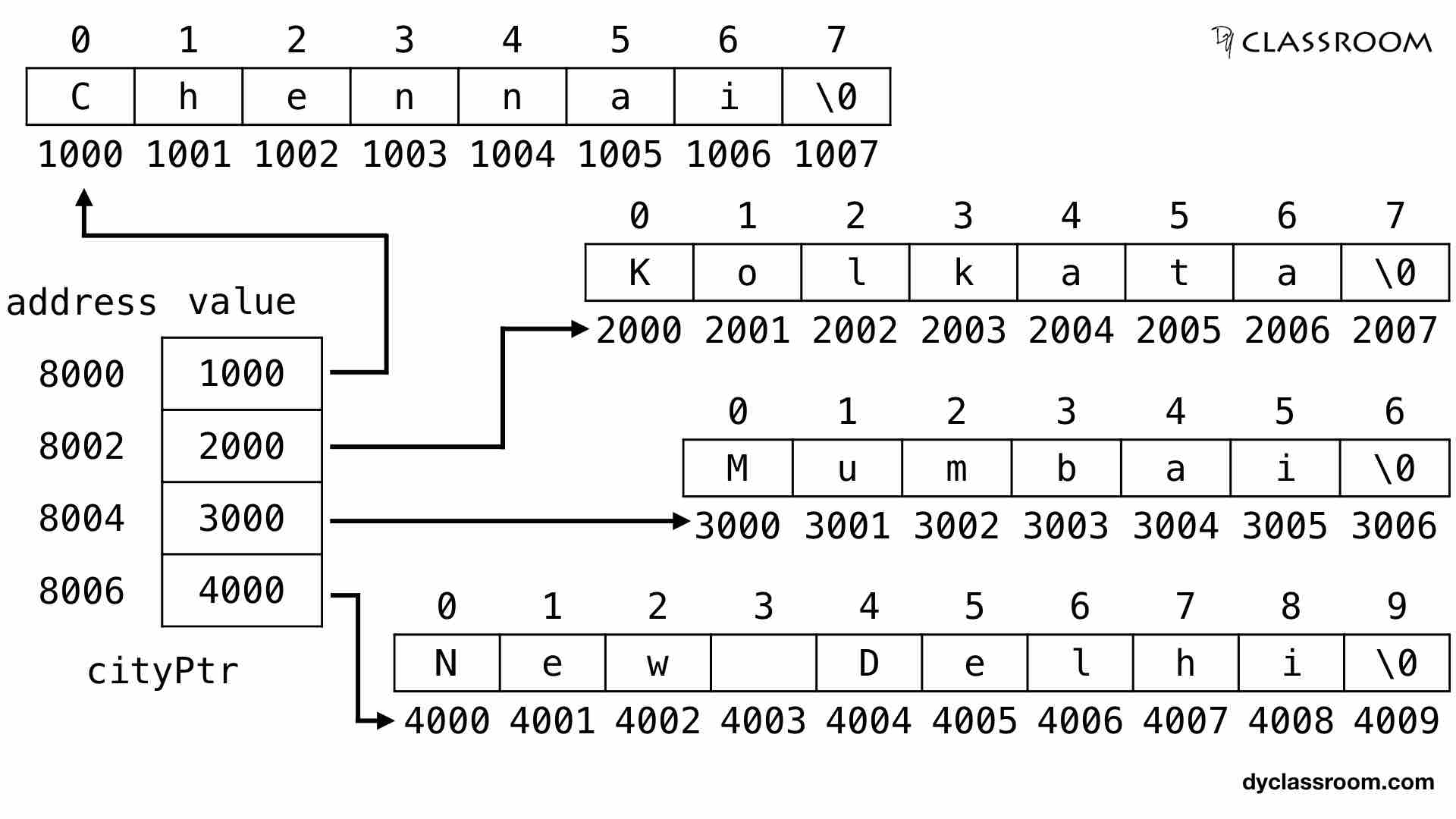The `cityPtr` pointer variable is allocated the memory address 8000 to 8007. Assuming integer address value takes 2 bytes space. So, each pointer gets 2 bytes.

Name of the cities are saved in locations 1000, 2000, 3000 and 4000.

## Accessing values pointed by array of pointers

To access and print the values pointed by the array of pointers we take help of loop as shown in the following example.

``````#include <stdio.h>

int main(void) {

// array of pointers
char *cityPtr = {
"Chennai",
"Kolkata",
"Mumbai",
"New Delhi"
};

// temporary variable
int r, c;

// print cities
for (r = 0; r < 4; r++) {
c = 0;
while(*(cityPtr[r] + c) != '\0') {
printf("%c", *(cityPtr[r] + c));
c++;
}
printf("\n");
}

return 0;
}
``````

Output:

``````Chennai
Kolkata
Mumbai
New Delhi
``````

In the above code we are using the `r` variable to access each row of the pointer. And we are using the `c` variable to access each character in a selected row.